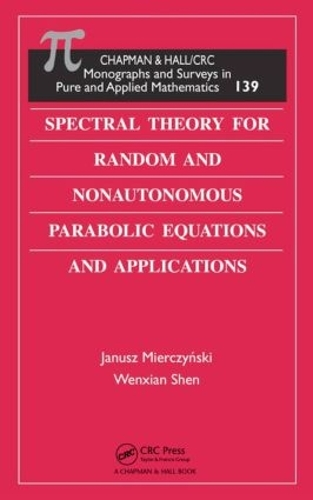•# Spectral Theory for Random and Nonautonomous Parabolic Equations and Applications - Monographs and Surveys in Pure and Applied Mathematics (Hardback)

(author), (author)
£96.99
Hardback 336 Pages / Published: 24/03/2008
• We can order this

Usually dispatched within 3 weeks

Providing a basic tool for studying nonlinear problems, Spectral Theory for Random and Nonautonomous Parabolic Equations and Applications focuses on the principal spectral theory for general time-dependent and random parabolic equations and systems. The text contains many new results and considers existing results from a fresh perspective.

Taking a clear, unified, and self-contained approach, the authors first develop the abstract general theory in the framework of weak solutions, before turning to cases of random and nonautonomous equations. They prove that time dependence and randomness do not reduce the principal spectrum and Lyapunov exponents of nonautonomous and random parabolic equations. The book also addresses classical Faber-Krahn inequalities for elliptic and time-periodic problems and extends the linear theory for scalar nonautonomous and random parabolic equations to cooperative systems. The final chapter presents applications to Kolmogorov systems of parabolic equations.

By thoroughly explaining the spectral theory for nonautonomous and random linear parabolic equations, this resource reveals the importance of the theory in examining nonlinear problems.

Publisher: Taylor & Francis Ltd
ISBN: 9781584888956
Number of pages: 336
Weight: 612 g
Dimensions: 235 x 159 x 23 mm